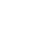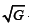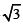info@biomedres.us   +1 (720) 414-3554
One Westbrook Corporate Center, Suite 300, Westchester, IL 60154, USA

# Biomedical Journal of Scientific & Technical Research

October, 2020, Volume 31, 3, pp 24261-24266Mini Review

Mini Review

# The Human Brain

### Paul T E Cusack*

Author Affiliations

23 Park Ave. Saint john, Canada

Received: October 13, 2020 | Published: October 29, 2020

Corresponding author: Paul TE Cusack, 23 Park Ave, Saint john, NB, Canada

DOI: 10.26717/BJSTR.2020.31.005108

#### Abstract

In this paper, we attempt to establish a mathematical foundation or set of equations for the human brain. We consider the brain as a black box, with internal energy and an input and output. The input is the sensory information. The internal energy is the hormones, motor activity and neurotransmitters energy. The output is the sum of the two. We can see for a plot of most of the equations that there is a mathematical solution to the human brain problem. Putting the brain and mind on a mathematical footing is beneficial toward predicting its behaviour.

Keywords: Brain Formulas; Neurotransmitters; Hormones; Senses; Mind as Black Box

#### Mini Review

In this paper, we use the equations of the brain and mind to develop a mathematical model for the brain. Using energy methods, we consider the input from the senses, the internal energy from the neurotransmitters and the output from the motor nerves, and the hormones. We begin with the cache of the formulas that have already been developed for the brain in previous papers.

The formulas necessary for understanding the human brain include:

1) t2 − t −1 = E

2) i = t2

3) V = iR

4) L = Lnt + c2

5) SE = E ⋅M

6) E = (1− Lnt)∧ 7

7) M = Lnt

8) E =1/ t

9) t = E2 + E − 2

10) E = dH = ST ds +V dp +Σμ N

11) Σ Senses =1/π +π + 4 ++=1 (Figure 1).

Figure 1: Plot of some of the Mind Equations.

There are hundreds of neurotransmitters. The most important ones are:

• Acetylcholine (Ach)

• Norepinephrine

• Serotonin

• Dopamine

• Phenylethylamine (PEA)

• GABA

• Glutamate

• Substance P

• CGRP

• Neuropeptide Y

• Glycine

t2 − t −1 = E

(1− Lnt)7

t=1.3888

i=t2

=1.38882

=1.929

(1-ln 1.3888)7

=0.0616

t2-t-1=

(0.616)2-(0.616)-1=-1.057=V

V=iR

-105.7=1.929R

R=0.548

M=ln t=Ln 1.3888=328

E=1/t=1/1.3888=1/7.2 =SE=(E·M)

SE=(-1/8 ·1/9)=1/72

t=E2-E-2

=(1/7.2)2+1/7.2-2

=654

### Enthalpy

E = dH = ST ds +V dp +Σμ N

dH = t(ΔQ / T) + 0 +Mc2

98=ΔQ+0+328c2

ΔQ=95.05=1/105.2~V

t2=ΔQ-dH

t2=ST-dH

i=ST -dH

V = iR

=(ST-dH)R

=(ST - dH)R

1.052=(ΔQ-dH)(548)

1.052/0.548=i=t2

=1.920

t=1.3855 Cf. 1.388

i=1.920

=(ΔQ-dH)

192.0=95.05-dH

dH =96.85

t2 − t −1 = E = E

(1)2-1-1=-2

E=-2=-L

L = Lnt + c3

2=Ln t+27

Ln t=-25

-Ln t=25

t=-3.218

t = E2 + E − 2

-3.218=E2+E-2

E=-2.22222; -1.22222

t=(1-SE/E)7

t=1-(-2/2.2222)=(1-1/(1/9)=-8

E=-1.25=Emin.

TE=M[0.15915]+ Delta Q

=1181..[.15915]+96.85

=115.6417=1/sin 60 deg.

We now consider the 5 most important neurotransmitters:

1) Dopamine C8H9N1O2 =138 amu

2) Serotonin C10H10N201=174 amu

3) GABA C4H6N102=100amu

4) Norepinephrine C8H10N1O3=168 amu

5) Glutamic Acid C5H7N1O4=145 amu

SUM=725 amu

M=Ln t

725=Ln t

t==2.0647

t^2-t-1=1.198~(1.2)

t^2-t-1=E

0.725^2-0.725-1=-1.998~(-1.2)

E = h x freq

=1/sin 60=6.626 t

12 pairs of cranial nerves and 31 pairs of Spinal Nerves.12/

{31/Ln31]=1.329

1.329 x2=2.658~2.66=SF

### Planck’s Equation

E = hυ

dH=E=1/sin 60°

2 x 1/sin 60°=2 (115.47)=2.3094~231

Ln (23.094)=8369=1/1.1947~1/12

### Sight and Sound

Sight

E = hυ

=(6.626)(380 nm-720nm)=2.517 - 4.770=2.25

Sound

E = hυ =(6.626)(20-20,000)=132.5 - 132,500=132.385

2.25-132.385=134.75

134.75 x 2=26.95~27=c3

L=2=(134.75)(2)=27 +Ln t

0=Ln t

t=1

0=Ln t

t=1

(Table 1).

M = Lnt

1=Ln t

t=e^1

E=1/e^1=e^-1

Input=Senses

V=iR=(1/c^2)(1)

105.7 (c^2)=R

R=950

Internal

t^2-t-1=E=1.500

t^2-t-2.5=0

t=21586; 115.83

Output

E = dH =1/ sin 60 =0.866

Input-Output=1/c^2-0.866=-0.7547

Input -Output +Internal=-0.755+1.5=745

TE = M[0.15915]+ deltasQ

0.745=M[0.15915]+96.05

M=1354~1350= Mass of Human Brain

M=Ln t

1.354=Ln t

t=26=Poisson’s Ratio

(Figure 2).

The sense of smell is used by animals to communicate, for mating, and for food detection and predator detection. Smell is tied in with the orbitofrontal cortex to be used in value judgement. Smell is the only sense that does not pass through consciousness but is directly hardwired to the limbic system. We can therefore smell before we are consciousness of it likely due to the flight or fight response. Its parameters are equal to the universal parameters including: Energy (Emin=-1.247); time (eigenvector= √3); Mass (=Ln t); the determinant |D|=4) and the gravitational constant (G=2/3=0.666) .

E = hυ

1/sin 60°=6.626(t)

Hormones =-1.247

V=iR=23.213=1/c2[π-1.247+√3)=403.5=Re

e4=546

e√3=549

GMP=-.1247

t=KE=1/2Mv2

=1/2(4)(1/2)

=1

e-(1/√3)=561

GMP=-.1247

TE = Mc2 +Mgh +1/ 2Mv2

=M{9+6.67)+1)

=M{16.67)

=M(10 x1/6)

=4(10x 1/6)

=40/6

=6.66=G

t=KE=1/2(4)(1/√2)2=1

M=4=Ln t

t=e4

Ln t=Ln e4=4=M

=e-1/√3

=561

GMP=-.12458

Ln (t)=Ln (2)=0.693

y=y’ y=2 t=1/2

Ln t=1/t

Ln (1/2)=-0.693=-Ln 2

Ln (1/t)=-Ln t

1/t=-t

1=-t2

t=√1=±1

t=1

2-Hydropropionic Acid

C3H603

=90 amu

M=Ln t

90=M=Ln t

t=1.2204

E=819.4

### Neurotransmitters

GABA 100amu

Glutamic Acid 145 amu

Aspartic Acid 133.103 amu

Glycine 75.067 amu

Σ 453.17 x 6.023 =2729 gm

M=Ln t=2729=Ln t

t=3.303

E=3.03

EL.A.- EN.T.=8194-3.03=0.816.4=√(2/3) = taste

Na+

K+

Cl-

Pr- 359

(NO3-;

H2S;

CO)

Ca+2

Vit. D 416

Σ= 775.1

+NT=725=1500.1

V=d/t

11.027=10m/t

t=10/11.027=906.8 =Minimum Time to recahrge~1 msec

P=i2R

i=t2=0.90682

P=0.906.84(950)

=676(950)

=6.422

P=Et

6.422=E(906.8)

E=708~1/√2

P=1/0.1557

1/√P=√(1/0.1557)=1.247=E

√P=1/1247=8.02=t

Universal Parametric Equation:

[csc600 ;3] = [E;t]

Brain:

[1;1]=[E;t]

ain niverse br =U

χ (1/ 3) =1/ sin 600

χ=2.0000

brain universe ψ t = t

ψ(1)=

ψ=3

χ/ψ=0.666=G

A plot of these two date points yields a linear equation:

m = rise / run = [(1/ sin 600 ) −1] / 3

=0.051578

~0.052

b=1-0.052=947

y=0.052x+948

E=0.052t+948

E=t=1

dE/dt=0.052

=(csc 60°-1)

t=[E-948]/0.052

dt/dt=1=[E-948]/0.052

E=899=c2

t2-t-1=-.052

t2-t-1.052 mV=0

t=1641;641

E=609;1.5

(Figure 3).

#### Conclusion

We see that the brain can be modelled by 11 formulas. They converse on: E=1; t-=1 and E=0; t=1; and E=-1,t=1.

Mini Review

# The Human Brain

### Paul T E Cusack*

Author Affiliations

23 Park Ave. Saint john, Canada

Received: October 13, 2020 | Published: October 29, 2020

Corresponding author: Paul TE Cusack, 23 Park Ave, Saint john, NB, Canada

DOI: 10.26717/BJSTR.2020.31.005108

#### Abstract

In this paper, we attempt to establish a mathematical foundation or set of equations for the human brain. We consider the brain as a black box, with internal energy and an input and output. The input is the sensory information. The internal energy is the hormones, motor activity and neurotransmitters energy. The output is the sum of the two. We can see for a plot of most of the equations that there is a mathematical solution to the human brain problem. Putting the brain and mind on a mathematical footing is beneficial toward predicting its behaviour.

Keywords: Brain Formulas; Neurotransmitters; Hormones; Senses; Mind as Black Box